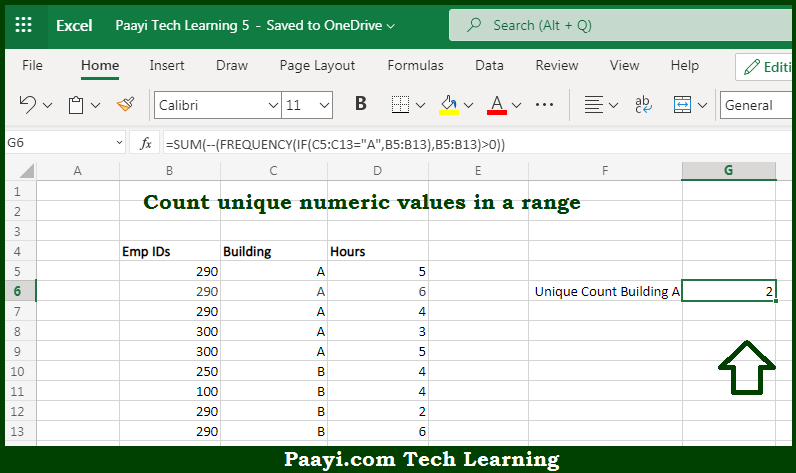# Learn How to COUNT Unique Numeric Values With a Criteria in Microsoft Excel

Written by | 0 Comments | 610 Views

In this article, you will learn how to COUNT various things in Microsoft Excel using a single or combination of functions and its purpose. You get to know how to COUNT Unique Numeric Values With Criteria and see the generic formula.

COUNT Unique Numeric Values With a Criteria in Microsoft Excel

The main purpose of this formula is to count unique numeric values in a range with criteria. Here we will learn how to count unique numeric values with criteria in the given range in Microsoft Excel. That implies, with the help of a formula based on the FREQUENCY, IF, and SUM function you can able to count unique numeric values in a range using given criteria. So, with the help of this formula, you can able to count unique numeric values in the given range with criteria.

General Formula to COUNT Unique Numeric Values With a Criteria

{=SUM(--(FREQUENCY(IF(criteria,values),values)>0))}

The Explanation for the COUNT Unique Numeric Values With a CriteriaSo we know that with the help of the given formula above you can able to count unique numeric values in a range with criteria. Here we will learn how to count unique numeric values with criteria in the given range in Microsoft Excel. As we know that usually, The Microsoft Excel FREQUENCY function returns a frequency distribution. The result is a summary table showing the frequency of numeric values, organized in "bins". We are using here it as a roundabout method to count unique numeric values. It should be noted that we are using the IF function for the criteria. So, with the help of this formula, you can able to count unique numeric values in a range with criteria.